# SAT Math Multiple Choice Question 87: Answer and Explanation

### Test Information

Question: 87

12. Luciano measured the amount of water that evaporated over a period of time from a container holding w ounces of water, where w is greater than 12. By the end of the first day, the cup had lost 2 ounces of water. By the end of the 7th day, the cup had lost an additional 8 ounces of water. By the end of the 11th day, the cup had lost half of the water that remained after the 7th day. Which of the following represents the remaining amount of water, in ounces, in Luciano's container at the end of the 11th day?

• A.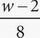• B.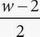- 10
• C.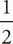w - 10
• D.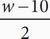D Whenever there are variables in the problem and in the answer choices, plug in. If w = 20, then Luciano's cup has 20 - 2 = 18 ounces at the end of day 1. At the end of 7 days, Luciano's cup would have 18 - 8 = 10 ounces. After 11 days, Luciano's cup would hold 10 - 5 = 5 ounces. Plug in 20 for w in the answer choices to see which answer is equal to the target number of 5. Choice (A) becomes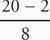=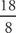= 2.25. This does not match the target number of 5, so eliminate (A). Choice (B) becomes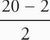- 10 =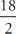- 10 = 9 - 10 = - 1. Eliminate (B). Choice (C) becomes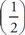(20) - 10 = 10 - 10 = 0. Eliminate (C). Choice (D) becomes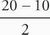=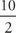= 5. This matches the target number; therefore, the correct answer is (D).